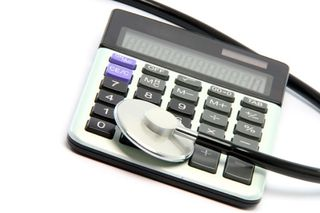# How Do Calculators Calculate?When you and I calculate two plus two, we imagine a number line, start at two and hop over a couple of spots to get to four (or at least we did until we memorized the answer). Simple — so simple, in fact, that "putting two and two together" is an analogy for deducing something obvious.

But what if you can't imagine a number line? What if you're a little, gray, button-covered box full of electrons? How, then, do you calculate that two plus two equals four?

The process itself is not so simple, but once you understand how this basic arithmetic operation works in a calculator, you can "put two and two together" as to how it performs much more complicated calculations.

First off, the input: when you press the "2" key, the button compresses a rubber pad below it, which in turn pushes an electrical wire into contact with another one, creating a circuit between them. Powered by a battery, electricity flows along the circuit to transistors.

Transistors are microscopic electrical switches that have two positions: on and off. The on position is represented by the number 1, and the off position by the number 0. (These are the famous 1s and 0sthat form the binary language of computers and calculators.) All numbers can be represented by a series of 1s and 0s. The number 2 — the one you keyed in for this calculation — sends electrons shooting into two transistors, turning one of them on and the other, off. The transistor configuration for "2" is thus "10."

Next, you press the "+" button. The rubber underneath it smashes two wires into contact, causing electricity to flow in such a way that the first input (the "2") gets stored, the addition function is signaled, and the calculator is ready for a new input. You press "2." Just like they did the first time, electric currents translate your "2" into binary code, by switching one transistor on and another off.

Finally, you press the "=" button. Now the calculator has the go-ahead to execute the previously entered "+" command. Two of its transistors (in the 10 configuration) correspond to the first "2" you pressed, and another pair of transistors, also in the 10 position, corresponds to the second number 2. Now the calculator must add those inputs together.

Calculators (and computers) combine inputs using electronic components called logic gates. As the name implies, a logic gate acts as a barrier in an electronic circuit; it takes in two electric currents, compares them and sends out a new current based on what it finds. There are several types of logic gates: AND, OR, NOT and XOR (exclusive OR). Together, the logic gates enable circuits to add, subtract, multiply and divide the numbers sent to them by transistors.

Take the AND gate: If both the signals that arrive at the gate are 1s, then AND sends out a 1. But if it receives a 1 and a 0, or two 0s, it sends out a 0.

OR sends out a 1 if either or both of its inputs is a 1, whereas XOR only outputs a 1 if its inputs are 1 and 0. The NOT gate switches the incoming signal: if its input is 1, its output is 0, and vice versa.

Logic gates are used to combine the information stored in the two pairs of transistors — 10 and 10 (representing 2 and 2). Even the simple operation of adding those numbers together requires a complicated array of logic gates, but here's the gist of the process:

The 1s are sent to an AND gate. The output is 1. The 1s are also sent to a XOR gate. Since both inputs are 1, the XOR gate outputs a 0. The original 0s (from 10 and 10) are also sent to AND and XOR gates, which both produce outputs of 0. In a process similar to "carrying the ten's place" in regular addition, the output from the 0s' AND gate gets combined with the output from the 1s' XOR gate: The 0 and 0 are sent through an OR gate, resulting in a 0. (There was nothing to carry.)

The final outputted signals are 1, 0 and 0. In binary code, 100 represents 4.

Additional logic gates "figure out" whether or not to darken the various sections used to form the shapes of numbers — typically composed of seven bars arranged as a digital figure eight — on the calculator screen. To make a 4, for example, the top bar does not need to be darkened. Sure enough, when 100 (representing 4) is sent through the array of logic gates associated with that top bar, the end result will be a 0 -- a "don't darken the bar" command.

All this circuitry happens in a flash, producing a 4 on the display seemingly instantaneously. To perform more complex calculations, such as multiplying or dividing huge numbers, a calculator performs a long series of simple additions and subtractions, all of which occur much like the above description of 2+2. Such calculations take more time, but still no more than the blink of an eye.